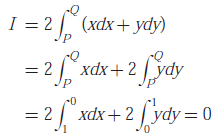# Engineering Mathematics - Online Test

Q1. The equation sin(z) = 10 has
Answer : Option A
Explaination / Solution:
No Explaination.

Q2. For real values of x , the minimum value of the function f (x) = exp(x) + exp(- x) is
Answer : Option A
Explaination / Solution: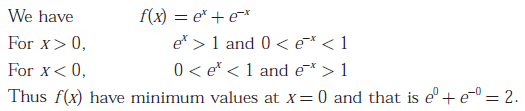Q3. Which of the following functions would have only odd powers of x in its Taylor series expansion about the point x = 0 ?
Answer : Option A
Explaination / Solution: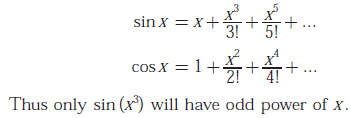Q4. Which of the following is a solution to the differential equationAnswer : Option B
Explaination / Solution: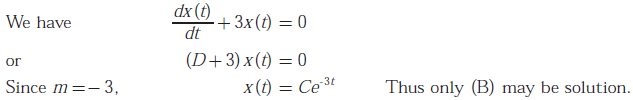Q5. The probability density function (pdf) of random variable is as shown below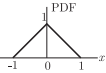The corresponding commutative distribution function CDF has the form
Answer : Option A
Explaination / Solution:
No Explaination.

Q6. The recursion relation to solve x = e-x using Newton - Raphson method is
Answer : Option C
Explaination / Solution: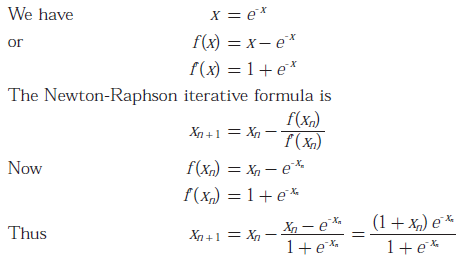Q7. The residue of the function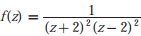at z = 2 is
Answer : Option A
Explaination / Solution: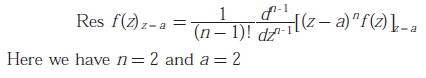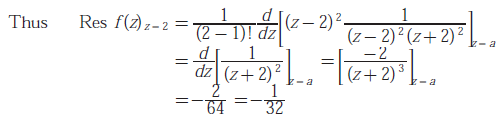Q8.
Consider the matrix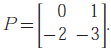The value of eis
Answer : Option D
Explaination / Solution: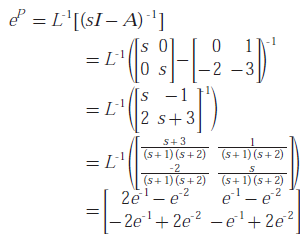Q9. In the Taylor series expansion of exp(x) + sin(x) about the point x = π, the coefficient of (x-π)2 is
Answer : Option B
Explaination / Solution: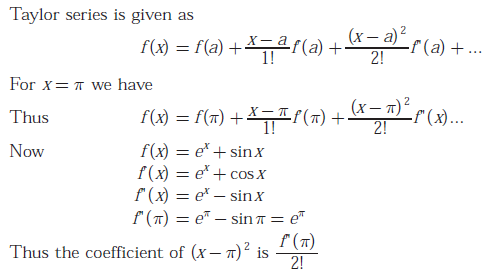Q10. Consider points P and Q in the x - y plane, with P = (1,0) and Q = (0,1). The line integral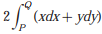along the semicircle with the line segment PQ as its diameter
Answer : Option B
Explaination / Solution: